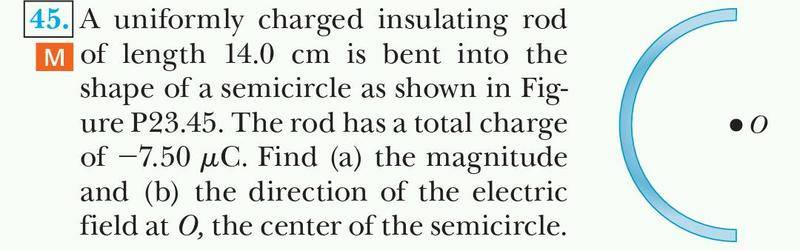# Finding electric field of a bent uniform charged rod.

freshcoast
1. Problem statement.2. Known equations.
E = k § dq/r^2

Lamda = Q/L

3. Attempt.

I am having trouble figuring out the dq in this situation. I know that lamda = Q/L, and since those 2 are the variables that are changing I take the derivative of them so it looks like

Lamda = dq/dL

I solve for dq giving me dq = lamda * dL

Which is where I am stuck. When I enter the new dq into the integral for the electric field, I do not know what to use for the r^2, I am aware that L=R(theta) but I am having trouble being able to manipulate R in terms of L since the only way is having a theta into the integral, but I cant integrate it because the bounds are going to be the length of the rod..

Any input is much appreciated, thanks!

Ampere
Try dq = lambda*r*d(theta) and integrating in polar coordinates. You will integrate over a half circle, i.e. from 0 to pi. R=r=constant because of the semicircle shape. You can use geometry to find the radius since the length of the rod is 1/2 the circumference of a full circle.

Tanya Sharma
Consider an element of length dl at angle θ .Now dq=λdl and dl=rdθ .The electric field due to this tiny part dl will be dE as shown in the figure.The component of dE in horizontal direction is dEsinθ and that in vertical direction is dEcosθ.This component dEcosθ is cancelled by a similar component of electric field due to element which is symmetric to this in the lower half of the semicircle.

So essentially we have,E=∫dEsinθ where dE=k(dq)/r2

#### Attachments

Last edited:
freshcoast
Hmm, I am still having trouble figuring out what R is,

from what Ampere is saying, I can set L = 2*pi*r, and since it is a semi circle, L = pi*r, and I can solve for R,

so far my integral looks like this

$$E = k∫λ r dθ /r2 (sinθ) (i)$$

after some simplification it ended up as

$$E = kλ/r * ∫sinθdθ (i)$$

lambda then turns into Q/L

$$E = [kq/L* R] * ∫sinθdθ (i)$$ integral bounds would be from 0 to pi

after integration I get,

$$E = kq/L*R * (-cos(pi) - (-cos(0)) (i)$$

$$E = kq/L * R * (-(-1) - (-(1)) (i)$$

$$E = 2kq/L*R$$

but when I plug everything I don't get the correct answer. the answer in the book is 2.16 N/C

btw I have another question about the direction, why is the direction of the force is pushing the point away instead of towards it, since the rod is negatively charged, doesn't that mean it should be an attraction force?

Tanya Sharma
Hmm, I am still having trouble figuring out what R is

R and r are the same thing.They are the distance of the point O from each tiny bit of the rod ,equal to the radius of the semi circular rod.

btw I have another question about the direction, why is the direction of the force is pushing the point away instead of towards it, since the rod is negatively charged, doesn't that mean it should be an attraction force?

Yes...the direction should be opposite to what i have shown in the figure.I didnt notice the rod was having negative charge.

Last edited:
freshcoast
R and r are the same thing.They are the distance of the point O from each tiny bit of the rod ,equal to the radius of the semi circular rod.

yes but I am having trouble finding the value of R given only L, since I know that R is related to L because length is equal to circumference of the circle, is it safe to say that R = L / pi ?

Tanya Sharma
∏r =L → r=L/∏

Tanya Sharma

Homework Helper
after some simplification it ended up as

$$E = kλ/r * ∫sinθdθ (i)$$

lambda then turns into Q/L

$$E = [kq/L* R] * ∫sinθdθ (i)$$ integral bounds would be from 0 to pi

.....
$$E = 2kq/L*R$$

but when I plug everything I don't get the correct answer. the answer in the book is 2.16 N/C

You multiplied by R instead of dividing by it, I am afraid. It should be $$E = \frac{2kq}{L*R}$$

ehild

freshcoast
Oh, that must be why, since I downloaded the pdf version of the book I guess it is not showing most of the answer, so the correct answer is 2.16 x 10^7 N/C, which is what I got, cool thanks!

Tanya Sharma
Well done :thumbs: